Latest Banking jobs   »

# Quantitative Aptitude Quiz For SBI PO/Clerk Mains 2022- 30th December

Q1. Prem gets an aggregate of 70% marks in five subjects in ratio 9 : 8 : 7 : 6 : 5. Maximum marks of each subject is same and 55% of maximum is needed to pass each exam. Then, in how many subjects did he pass the examination?
(a) 3
(b) 2
(c) 5
(d) 1
(e) 4

Q2. The average price of a TV, washing machine & refrigerator is Rs 5000 less than average price of TV & washing machine. The amount by which the price of TV is more than that of washing machine is same as amount by which price of refrigerator is less than that of washing machine. Find difference between average price of TV & washing machine & average price of washing machine & refrigerator.
(a) Rs 10000
(b) Rs 30000
(c) Rs 5000
(d) Cannot be determined
(e) None of these

Q3. Anshul deposited two parts of a sum of Rs. 25000 in different banks at the rates of 15% per annum and 18% per annum respectively at SI. In one year he got Rs. 4050 as the total interest. What was the amount deposited at the rate of 18% per annum?
(a) Rs. 9000
(b) Rs. 18000
(c) Rs. 15000
(d) Rs. 10000
(e) Rs. 12000

Q4. A watch dealer usually sells watches for Rs.2350 per watch. Once he gave two successive discounts of 15% and 25% while selling a watch to a customer. But he charged an additional 8% on the net sale price from the customer. By what percent is the new selling price less than the original selling price?
(a) 28.45%
(b) 29.25%
(c) 30.45%
(d) 31.15%
(e) 24.25%

Q5. P and Q started a business by investing Rs. 45,000 and Rs.54,000 respectively. After four months R joined the business with a capital of Rs. 30,000. After two more months Q left the business with his capital. At the end of the year P got a share of Rs. 13,500 in the profit. What is the total profit earned?
(a) Rs 26800
(b) Rs 27600
(c) Rs 28600
(d) Rs 29200
(e) Rs 32300

Q6. A sold an article to B at 20% profit and B further sold it to C at 20% loss. C then sold it to D at 25% profit. If difference of cost price of article for A & C is Rs.17, then find the amount at which C sold the article to D.
(a) Rs.510
(b) Rs.720
(c) Rs.810
(d) Rs.640
(e) Rs.450

Q7. An ice cream box full of ice cream is in the form of cuboid, having 54cm breadth, 30cm height and 77cm length. Ice cream from the cuboidal box is served in the cone. Radius of cone is 425% more than its height. If ratio of height of the cone to cuboid is 1 : 5, then find the number of cones required to serve the full quantity of ice cream of cuboidal box.
(a) 25
(b) 10
(c) 30
(d) 15
(e) 20

Q8. There are 3 vessels – A, B & C, each containing 600ml solution of milk and water. Vessel – A, B & C contains 75% milk, 45⅚% water and 66⅔% milk respectively. 100 ml mixture is taken out from A and poured into B, then 175 ml mixture is taken out from B and poured into C and then 155 ml mixture is taken out from C and poured into A. Find ratio of milk to water in A in the end.
(a) 30 : 13
(b) 19 : 7
(c) 8 : 3
(d) 95 : 36
(e) None of the above.

Q9. Three cards are drawn randomly from a pack of 52 cards. Find the probability of getting at least two ace cards.
(a) 7/325
(b) 3/85
(c) 73/5525
(d) 457/5525
(e) 3/65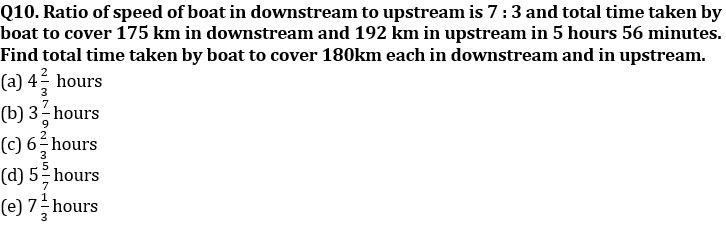Q11. A certain number of boys take 1.5 days less than a certain number of men to complete a task. Work done by four men in one day is same as the work done by six boys in one day. Which one of the following number of men and number of boys can satisfy the condition given above?
(i) 3 , 6
(ii) 6, 12
(iii) 8, 3
(iv) 10, 24
(a) only (ii)
(b) only (ii) and (iii)
(c) only (i) and (iii)
(d) All of the above
(e) only (i), (ii) and (iv)

Direction (12 – 13) : Veer invested Rs. 3400 in a scheme ‘P’ for two years which offered S.I. at the rate of R% per annum and gets total interest of Rs. 1088. There is also another scheme ‘Q’, which offered CI annually at the rate of (R – 6) %.

Q12. If a man invested Rs. (2000 + x) in scheme ‘P’ for 2 years and Rs. (1600 + 3x) in scheme ‘Q’ for 2 years and interest gets from scheme P is Rs. 56 more than interest gets from scheme Q, then find total amount invested in scheme ‘Q’?
(a) 800 Rs.
(b) 1000 Rs.
(c) 1600 Rs.
(d) 4000 Rs.
(e) 2000 Rs.

Q13. A man invested an amount in the ratio of 3 : 2 at the rate of (R/2-0.5)% & (R – 8)% respectively at simple interest. If bigger amount invested for two years and man gets interest in the ratio of 15 : 16, then find smaller amount invested for how many years?
(a) 2 years
(b) 3 years
(c) 5 years
(d) 7 years
(e) 11 years

Q14. The ratio between the radius of two hemispherical solid tin pieces is 2 : 3 and difference between volumes of both of the the pieces is 6688/21 cm³. These two hemispheres are melted and recast into a cylindrical vessel and used 592/3 π cm³ extra tin material for polishing the vessel. If ratio between height & radius of cylindrical vessel is 3 : 4, then find the total surface area of cylindrical vessel?
(a) 724 cm²
(b) 176 cm²
(c) 704 cm²
(d) 744 cm²
(e) None of these

Q15. A bag contains some red and some blue balls in the ratio of 4 : 5. If four red balls are added in the bag and one ball is taken out at random from the bag, then probability of that ball being Red is 6/11. Five balls are taken out from the bag, find probability so that the red balls left in the bag are maximum?
(a) 7/13
(b) 8/13
(c) 6/13
(d) 5/13
(e)None of these

Solutions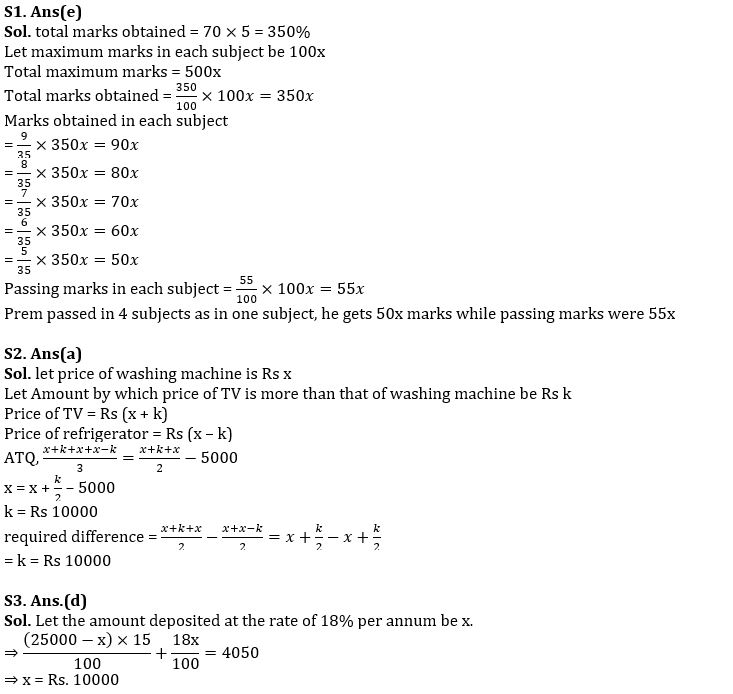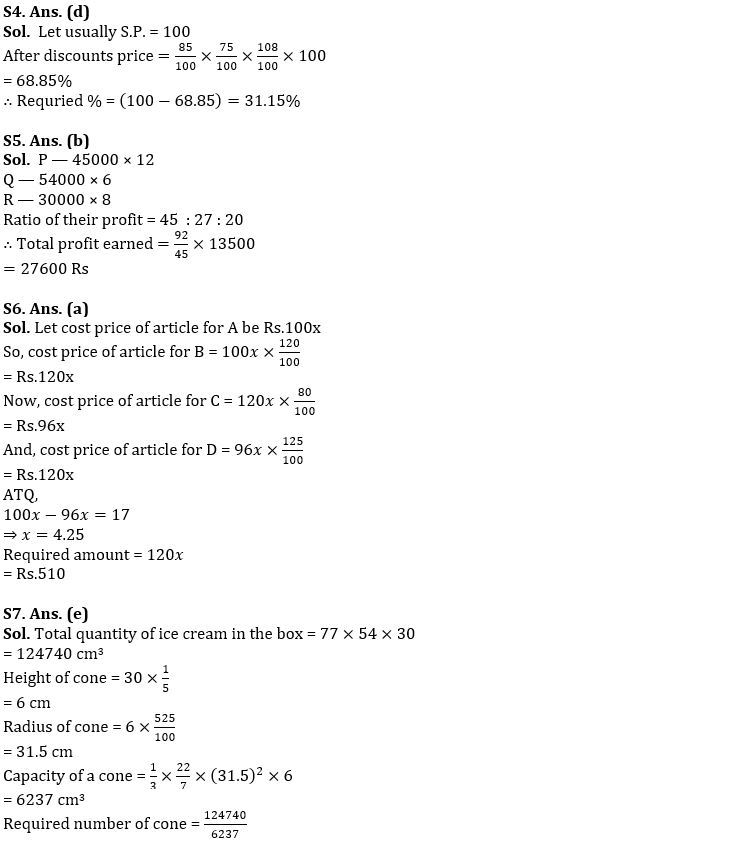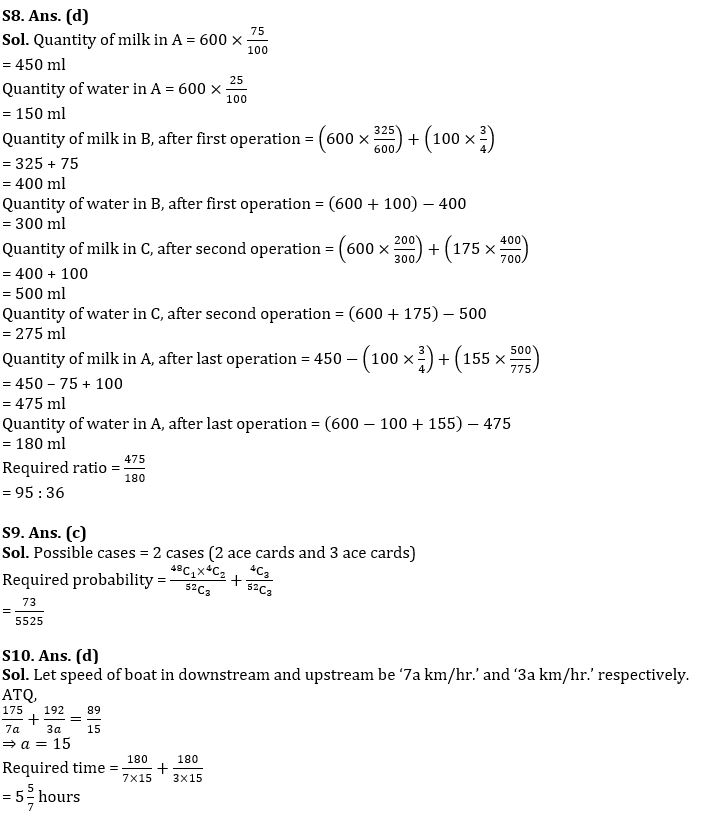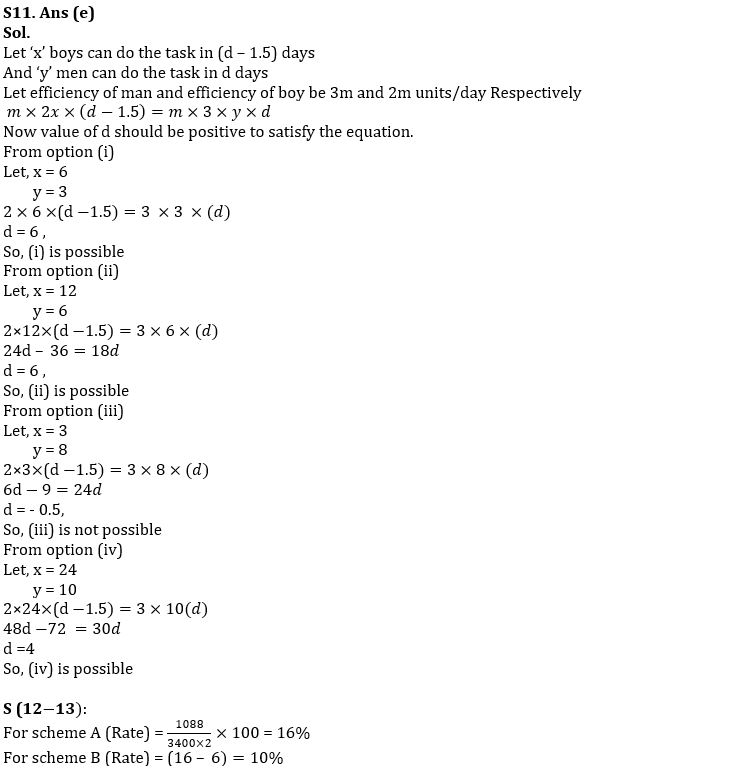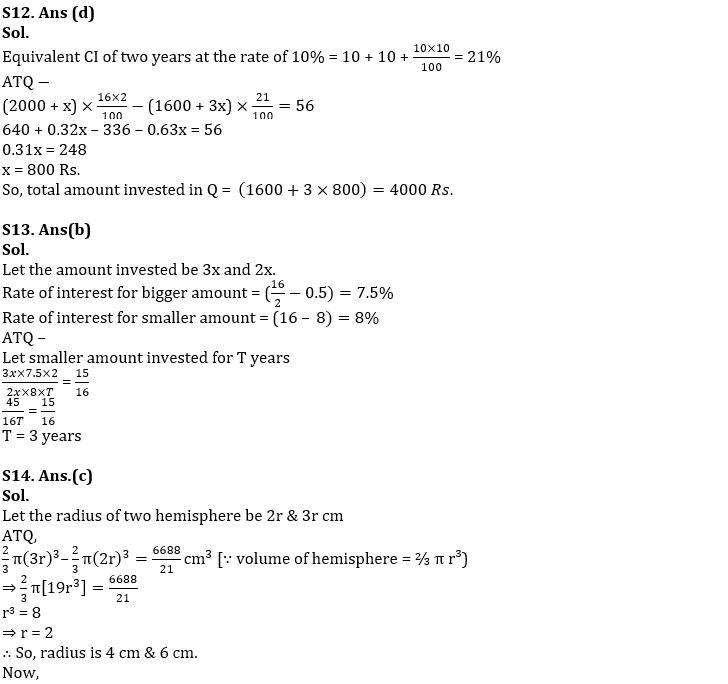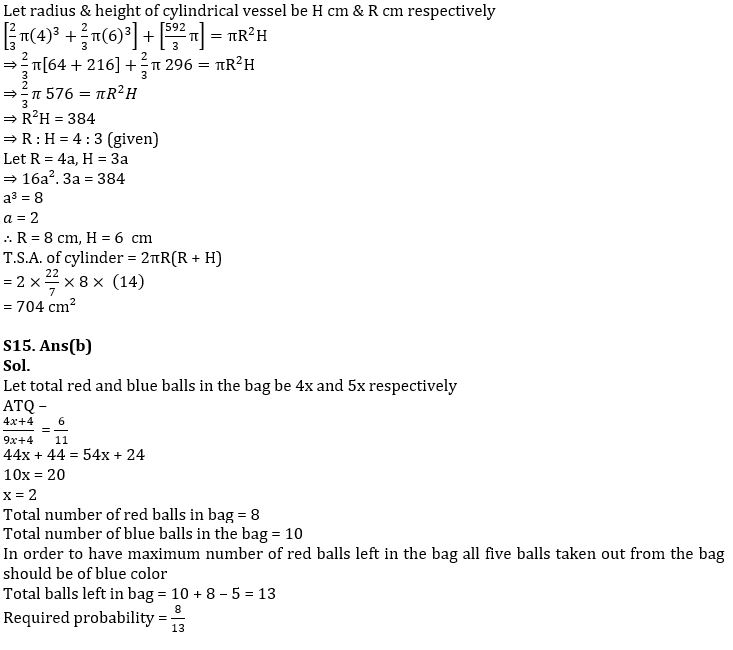#### Congratulations!Union Budget 2023-24: Free PDF